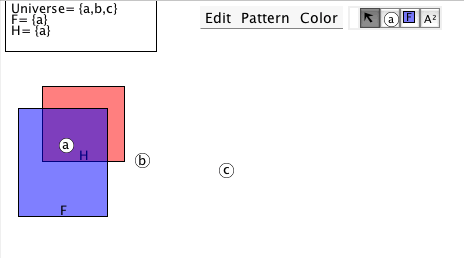# Tutorial 17: The semantics of quantifiers

Logical System

10Software

### The Tutorial

We will certainly wish to discuss the truth and falsity of formulas with quantifiers in them.

Interpretation 1

Universe= {a,b}
F={a}

and an atomic formula with a variable in it, Fx, say. Is this formula true in Interpretation 1? Well, the formula says 'x is F' and we know that x is supposed to be the name of something but the trouble is that we do not know what it is the name of. This makes quite a bit of difference-- if x is the name of the object a in the Universe then the formula is true, but if x is the name of the object b in the Universe then the formula is false. What has to insisted on here is some direction as to what x is supposed to name. x has to be valued. We have a notation for this, an example of it is

Fx[a/x]

and this is read 'Fx with x naming a' or 'Fx with x valued to be a'. Now, Fx[a/x] is true in Interpretation 1 whereas Fx[b/x] is false in Interpretation 1.

Our earlier work on Interpretations and Truth has to be extended to take in Valuations. What a Valuation should do is to assign to every variable in the language an entity in the Universe for that variable to denote. We have as variables m,n...z so a Valuation should give a value for all 14 of these. But, it is rare for a formula to contain more than a few variables; and whether a formula is true in a valuation depends only on the variables that the formula contains; so we can scrape by with valuing only the free variables that the formula contains. For example, take Gx... from a purist's point of view we should value every variable in the language, we should be looking at Gx[a/m,b/n,a/o,b/p,a/q,b/r,a/s,b/t,a/u,b/v,a/w,b/x,a/y,b/z] but Gx does not contain any of the variables m,n,o,p,q,r,s,t,u,v,w,y,z so we can squeek by with Gx[b/x].

A formula that has a Universal Quantifier as its main connective has its truth value assigned in an obvious way. Consider again

Interpretation 1 Universe= {a,b}
F={a}

and the formula

(∀x)(Fx).

The formula says 'whatever x you chose, x is F' and this is false in Interpretation 1 because if you chose b, b is not F, that is Fx[b/x] is false. (What you have to do mentally is to take the scope of the quantified formula and look also at the Universe of the Interpretation and run through Fx[a/x], Fx[b/x], ... for all the objects in the Universe and see if they are all suitable.)

A formula that has a Existential Quantifier as its main connective also has its truth value assigned in an obvious way. Consider again

Interpretation 1 Universe= {a,b}
F={a}

and the formula

(∃x)(Fx).

The formula says 'There is an x such that x is F' and this is true in Interpretation 1 because if you chose a, a is F, that is Fx[a/x] is true. (What you have to do mentally is to take the scope of the quantified formula and look also at the Universe of the Interpretation and run through Fx[a/x], Fx[b/x], ... for all the objects in the Universe and see if at least one will do the job.)

Some further concepts... If an occurrence of a variable occurs within the scope of a quantifier that uses that variable, that occurrence is said to be bound. Otherwise the occurrence is free. The need to value arises with formulas with free variables.

## Exercise to accompany Predicate Tutorial 7

The Interpretation in Predex7 shows an Interpretation similar to thisthe applet should display this, but if it does not just draw a similar one.

### Exercise 1 (of 3)

Interpretations Applet